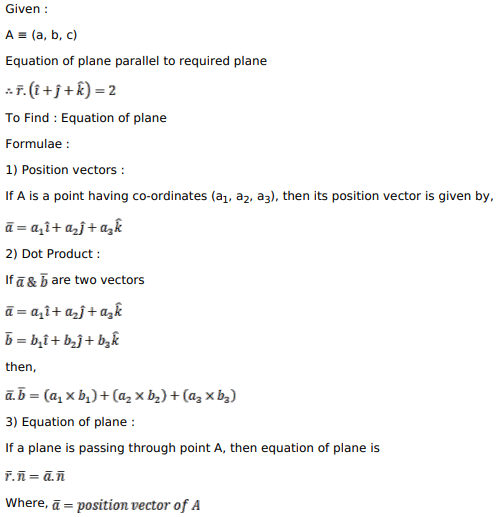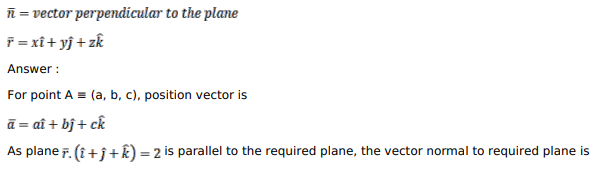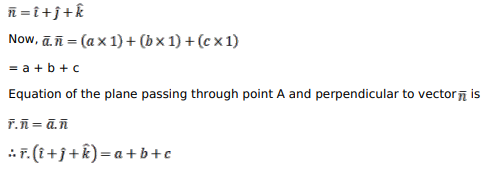# Write the equation of the plane passing through the point

Question:

Write the equation of the plane passing through the point $(\mathrm{a}, \mathrm{b}, \mathrm{c})$ and parallel to the plane $\bar{r} \cdot(\hat{i}+\hat{j}+\hat{k})=2$.

Solution: